# E-PolyLearning

 61. Choose the figure which is different from the rest.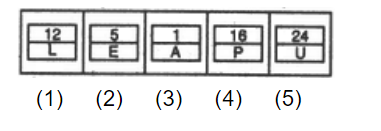a. 1 b. 2 c. both 3 and 4 d. 5

 62. Choose the figure which is different from the rest.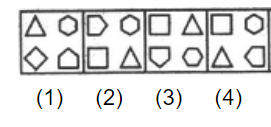a. 1 b. 2 c. 3 d. 4
 63. Choose the figure which is different from the rest.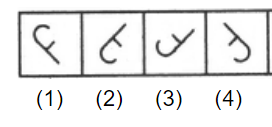a. 1 b. 2 c. 3 d. 4
 64. Choose the figure which is different from the rest.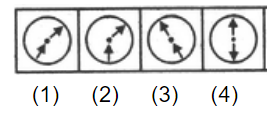a. 1 b. 2 c. 3 d. 4
 65. Choose the figure which is different from the rest.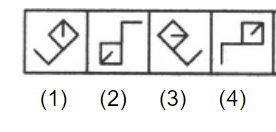a. 1 b. 2 c. 3 d. 4